I'm going to show some results of numerical simulations of earthquake nucleation that we've been doing where we've included the effect of thermal pressurization. I going to show you that thermal pressurization is actually important during the earthquake nucleation, before seismic slip.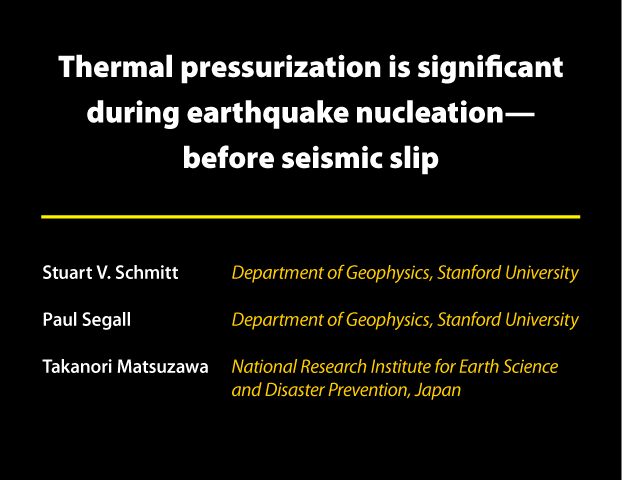For those of you who are just now joining the session, here's a brief description of thermal pressurization. Friction on a sliding fault generates heat, causing pore fluid to expand. If the rate of heat production causes a pressure build-up that can't be outpaced by diffusion, effective normal stress decreases, weakening the fault. This is an old idea that has been considered in by many researchers. Only the last one in the list has considered thermal pressurization in the context of earthquake nucleation.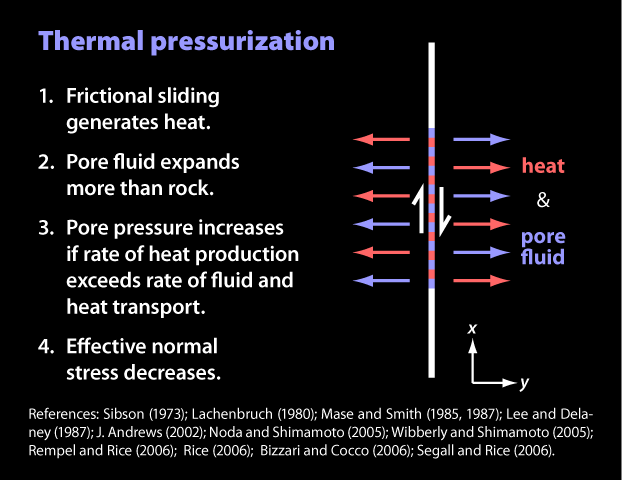Here are the questions I will address. One, at what critical slip speed does thermal pressurization dominate frictional weakening? Two, how does that critical slip speed depend on hydraulic diffusivity near the fault? Three, how does thermal pressurization influence earthquake nucleation?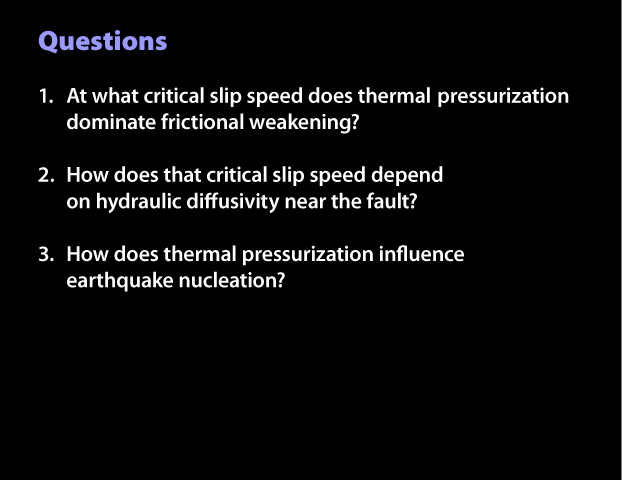Here are some of the governing equations for these simulations. The first one is our equation of motion. It is, simply, stress minus frictional weakening equals radiation damping. We're using rate and state friction, and in this work we have been using both the aging and slip evolution laws.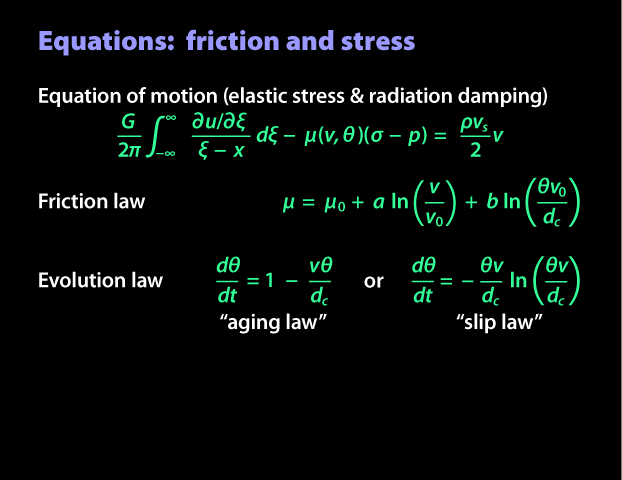Next are the equations for fluid and heat transport. We consider a planar fault model. That is, one where the the shear zone thickness is zero. Because of that, heating on the fault is a boundary condition. Pore pressure diffusion is next, with thermal pressurization as a source term. Lambda is the thermal pressurization factor that I define below. Jim Rice showed in his paper last year that the pressure change on the fault is proportional to the temperature change, following this equation. Lambda is a function of thermal expansivities of the fluid and pores, in the numerator, and their compressibilities in the denominator. Because of this relationship, we only need to compute the temperature field to know the pore pressure on the fault. I also should note that in the coupling term (the term relating T to p), the widest-ranging parameter is probably the hydraulic diffusivity. With reasonable estimates for all of the parameters, the pressure-temperature coupling is an MPa per Kelvin.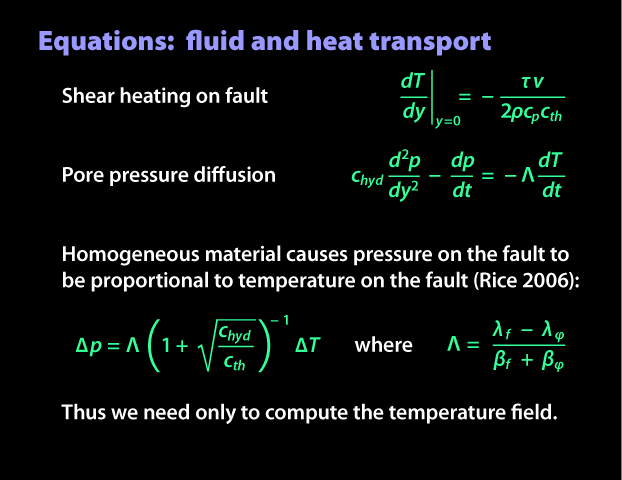We do our simulations in 2D, where the fault is a line. We do finite difference calculations for diffusion. You can see the diffusion in this plot. We are showing some snapshots of temperature profiles normal to the fault at various times. Increasing brightness indicates later time. Our finite difference grid is automatically refined if accuracy demands it. We drive our simulations by forcing a creep velocity at the ends of the fault. We start them above steady state (d&theta/dt > 0) with a slightly higher velocity at the middle so we can control where nucleation occurs.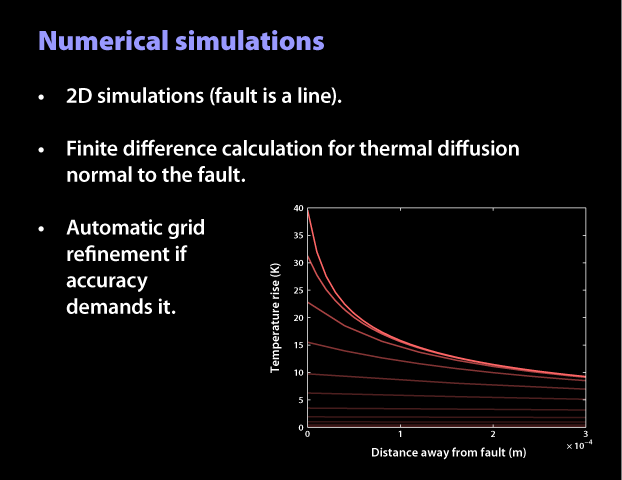And now some results. In this slide and the one that follows, I am showing you profiles along the fault. The left column in green is with thermal pressurization turned off and the right column in red is with thermal pressurization turned on. The top row is snapshots of log-scale slip speed and the bottom row is snapshots of frictional resistance. The snapshots are selected such that they represent half-decades of maximum slip speed. They are not linear in time. The simulations were turned off at a tenth of a meter per second. Frictional parameters are up here. Most importantly, a/b = 0.8 and hydraulic diffusivity is 10-6 m2/s. These results are for the aging law. With thermal pressurization turned off, the nucleation zone looks like a slowly expanding crack. This is the result we expect based on the results of the 2005 Rubin and Ampuero paper. With thermal pressurization turned on, notice that the slip speed profile follows the same track up to a critical slip speed, then the nucleation zone contracts. We see the same thing in the frictional resistance. Just as the slipping zone contracts, so does this decrease in frictional resistance.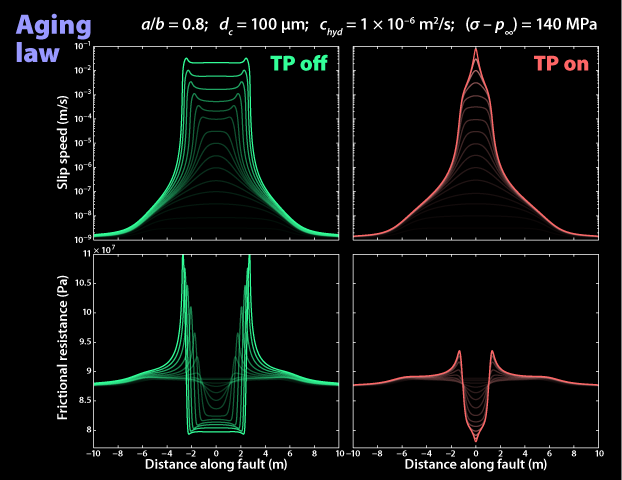And here are the results for the slip law. Other than the evolution law, we used the same frictional parameters. With thermal pressurization off, the slip profile is more pulse-like. And just like with the aging law, the slip profile with thermal pressurization on shows that it follows the same track up to a critical velocity. And looking at the frictional resistance, we see that weakening moves to the middle with thermal pressurization on. This is of course where the heat generation is the highest. From here on, we are going to look at plots of quantities at the middle of fault.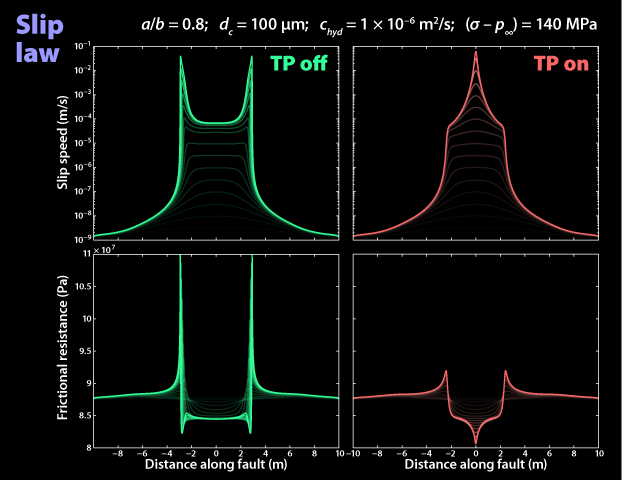We devised a way to quantify that critical velocity. It is the velocity when the rate of change of the pressure effect exceeds the rate of change of the friction effect. Here it is for the aging law simulation from two slides ago. You can see that it's about 10-4 m/s for this simulation. That's well under seismic slip speeds. You might notice that rate of change of friction changes sign—that it moves toward being a strengthening effect. This is because of the so-called direct effect, or the a term, which is directly proportional to log velocity. What's happening is the pressure weakening is so strong that its driving the fault faster than the state can't evolve and keep up.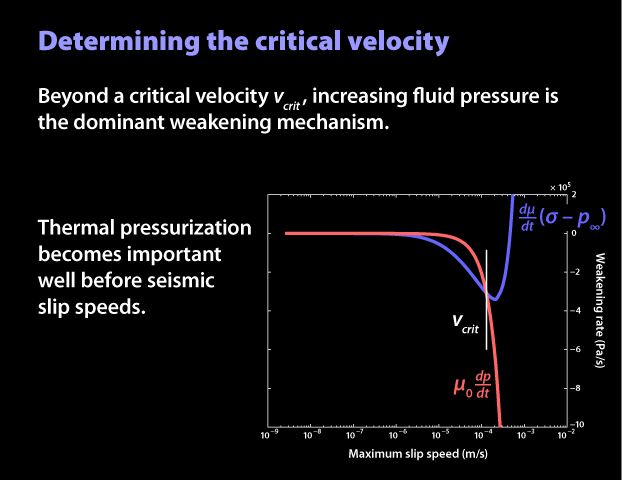There are two things I want to cover in this slide. First is the relationship of critical velocity and hydraulic diffusivity. Jim Rice tabulated permeability data from some studies of fault core rocks and calculated a diffusivity. He found a rather constrained range of values, but given the wide range of rock permeabilities, we wanted to study the effect of thermal pressurization over a wider range. In this case, 10-7 m2/s up to 10-3 m2/s. We ran eight simulations using these frictional parameters and plot the critical velocity here in cyan. As we expect, the critical velocity increases with increasing hydraulic diffusivity. The other thing I want to show you is a comparison to an analytical estimate of critical velocity. In their paper last year, Paul Segall and Jim Rice present this expression as a conservative estimate, or upper bound, of critical velocity. They compare a case without thermal pressurization to one where it is present, but does not include the temperature-pressure-weakening feedback cycle. Here are their estimates in green. They are indeed an upper bound.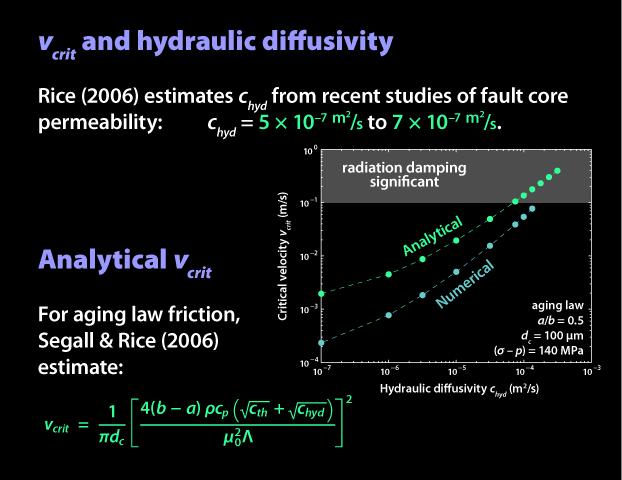And finally, I need to show you how maximum slip speed evolves in time. This plot shows it in scale. Note the time axis is only 1000 seconds at the end of 31,000. This time is not particularly meaningful because it is just the time to nucleation since the initial condition of the simulation. The green line is log slip speed with thermal pressurization turned off. The red line is with it on. The important thing to note is that thermal pressurization only slightly advances the time-to-instability.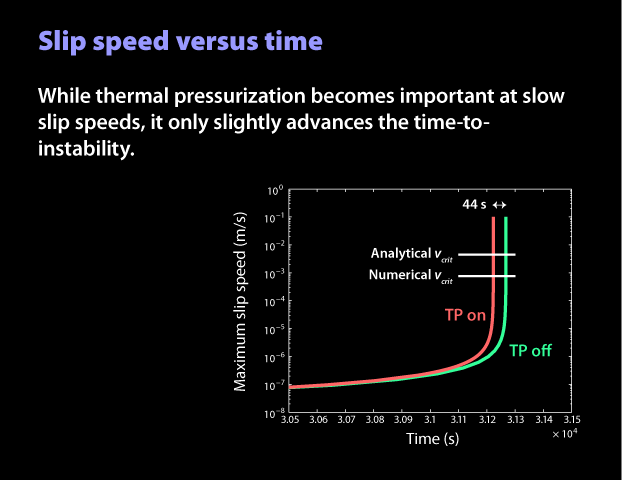Here's what we can conclude. One, thermal pressurization is important during nucleation. This may be inconsistent with hypothesized changes in behavior at intermediate earthquake magnitudes that call upon thermal pressurization as their mechanism. Two, thermal pressurization causes the nucleation zone to contract for both evolution laws. Thus, detecting precursory strain before earthquakes is even more challenging, and is perhaps even impossible. And three, thermal pressurization does not significantly affect the time-to-instability. Thus, models of seismicity rate changes based on that time will not really be affected by thermal pressurization.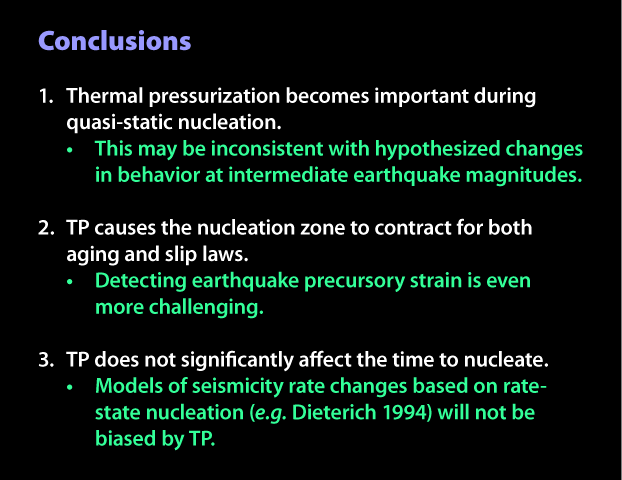PDF of the slides only